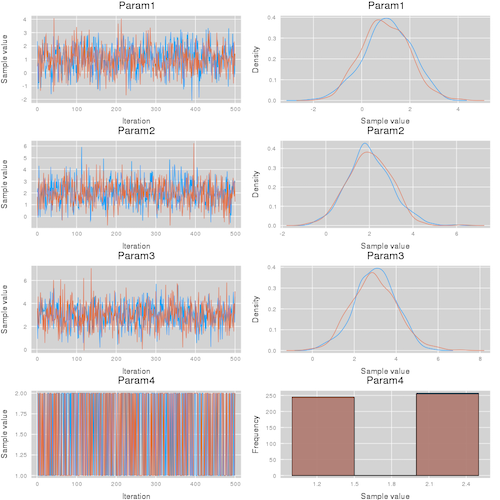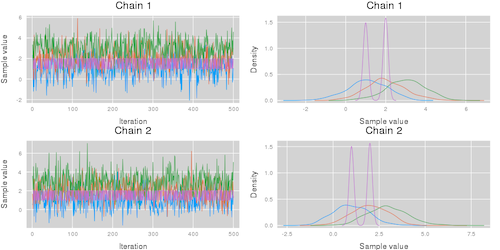96

15

14

18

# MCMCChains.jlImplementation of Julia types for summarizing MCMC simulations and utility functions for diagnostics and visualizations.

## Example

The following simple example illustrates how to use Chain to visually summarize a MCMC simulation:

``````using MCMCChains
using StatsPlots

theme(:ggplot2)

# Define the experiment
n_iter = 500
n_name = 3
n_chain = 2

# experiment results
val = randn(n_iter, n_name, n_chain) .+ [1, 2, 3]'
val = hcat(val, rand(1:2, n_iter, 1, n_chain))

# construct a Chains object
chn = Chains(val)

# visualize the MCMC simulation results
p1 = plot(chn)
p2 = plot(chn, colordim = :parameter)
``````

This code results in the visualizations shown below. Note that the plot function takes the additional arguments described in the Plots.jl package.

Summarize parameters  Summarize chains
`plot(chn; colordim = :chain)` `plot(chn; colordim = :parameter)`## Manual

### Chains type

``````# construction of a Chains object with no names
Chains(
val::AbstractArray{A,3};
start::Int=1,
thin::Int=1,
evidence = 0.0,
info=NamedTuple(),
)

Chains(
val::AbstractArray{A,3},
parameter_names::AbstractVector,
name_map = (parameters = parameter_names,);
start::Int=1,
thin::Int=1,
evidence = 0.0,
info=NamedTuple(),
)

# Indexing a Chains object
chn = Chains(...)
chn_param1 = chn[:,2,:] # returns a new Chains object for parameter 2
chn[:,2,:] = ... # set values for parameter 2
``````

### Parameter Names

Chains can be constructed with parameter names, like so:

``````# 500 samples, 5 parameters, two chains.
val = rand(500,5, 2)

chn = Chains(val, ["a", "b", "c", "d", "e"])
``````

By default, parameters will be given the name `:param_i`, where `i` is the parameter number.

### Rename Parameters

Parameter names can be changed with the function `replacenames`, which accepts a `Chains` object and pairs of old and new parameter names. Note that `replacenames` creates a new `Chains` object that shares the same underlying data.

``````chn = Chains(
rand(100, 5, 5),
["one", "two", "three", "four", "five"],
Dict(:internals => ["four", "five"])
)

# Set "one" and "five" to uppercase.
chn2 = replacenames(chn,  "one" => "ONE", "five" => "FIVE")

# Alternatively you can provide a dictionary.
chn3 = replacenames(chn, Dict("two" => "TWO", "four" => "FOUR"))
``````

### Sections

Chains parameters are sorted into sections that represent groups of parameters. By default, every chain contains a `:parameters` section, to which all unassigned parameters are assigned to. Chains can be assigned a named map during construction:

``````chn = Chains(val,
["a", "b", "c", "d", "e"],
Dict(:internals => ["d", "e"]))
``````

The `set_section` function returns a new `Chains` object:

``````chn2 = set_section(chn, Dict(:internals => ["d", "e"]))
``````

Any parameters not assigned will be placed into `:parameters`.

Calling `display(chn)` provides the following output:

``````Chains MCMC chain (500×5×2 Array{Float64,3}):

Iterations        = 1:500
Thinning interval = 1
Chains            = 1, 2
Samples per chain = 500
parameters        = a, b, c
internals         = d, e

Summary Statistics
parameters      mean       std   naive_se      mcse         ess      rhat
Symbol   Float64   Float64    Float64   Float64     Float64   Float64

a    0.4930    0.2906     0.0092    0.0095   1044.0585    1.0030
b    0.5148    0.2875     0.0091    0.0087    992.1013    0.9984
c    0.5046    0.2899     0.0092    0.0087    922.6449    0.9987

Quantiles
parameters      2.5%     25.0%     50.0%     75.0%     97.5%
Symbol   Float64   Float64   Float64   Float64   Float64

a    0.0232    0.2405    0.4836    0.7530    0.9687
b    0.0176    0.2781    0.5289    0.7605    0.9742
c    0.0258    0.2493    0.5071    0.7537    0.9754
``````

Note that only `a`, `b`, and `c` are being shown. You can explicity retrieve an array of the summary statistics and the quantiles of the `:internals` section by calling `describe(chn; sections = :internals)`, or of all variables with `describe(chn; sections = nothing)`. Many functions such as `plot` or `gelmandiag` support the `sections` keyword argument.

### Groups of parameters

By convention, MCMCChains assumes that parameters with names of the form `"name[index]"` belong to one group of parameters called `:name`. You can access the names of all parameters in a `chain` that belong to the group `:name` by running

``````namesingroup(chain, :name)
``````

If the chain contains a parameter of name `:name` it will be returned as well.

The function `group(chain, :name)` returns a subset of the chain `chain` with all parameters in the group `:name`.

### The `get` Function

MCMCChains provides a `get` function designed to make it easier to access parameters `get(chn, :P)` returns a `NamedTuple` which can be easy to work with.

Example:

``````val = rand(500, 5, 1)
chn = Chains(val, ["P", "P", "P", "D", "E"]);

x = get(chn, :P)
``````

Here's what `x` looks like:

``````(P = (Union{Missing, Float64}[0.349592; 0.671365; … ; 0.319421; 0.298899], Union{Missing, Float64}[0.757884; 0.720212; … ; 0.471339; 0.5381], Union{Missing, Float64}[0.240626; 0.987814; … ; 0.980652; 0.149805]),)
``````

You can access each of the `P[. . .]` variables by indexing, using `x.P`, `x.P`, or `x.P`.

`get` also accepts vectors of things to retrieve, so you can call `x = get(chn, [:P, :D])`. This looks like

``````(P = (Union{Missing, Float64}[0.349592; 0.671365; … ; 0.319421; 0.298899], Union{Missing, Float64}[0.757884; 0.720212; … ; 0.471339; 0.5381], Union{Missing, Float64}[0.240626; 0.987814; … ; 0.980652; 0.149805]),
D = Union{Missing, Float64}[0.648963; 0.0419232; … ; 0.54666; 0.746028])
``````

Note that `x.P` is a tuple which has to be indexed by the relevant index, while `x.D` is just a vector.

### Convergence Diagnostics functions

#### Discrete Diagnostic

Options for method are `[:weiss, :hangartner, :DARBOOT, MCBOOT, :billinsgley, :billingsleyBOOT]`

``````discretediag(c::Chains; frac=0.3, method=:weiss, nsim=1000)
``````

#### Gelman, Rubin, and Brooks Diagnostics

``````gelmandiag(c::Chains; alpha=0.05, mpsrf=false, transform=false)
``````

#### Geweke Diagnostic

``````gewekediag(c::Chains; first=0.1, last=0.5, etype=:imse)
``````

#### Heidelberger and Welch Diagnostics

``````heideldiag(c::Chains; alpha=0.05, eps=0.1, etype=:imse)
``````

#### Raftery and Lewis Diagnostic

``````rafterydiag(c::Chains; q=0.025, r=0.005, s=0.95, eps=0.001)
``````

#### Rstar Diagnostic

Rstar diagnostic described in https://arxiv.org/pdf/2003.07900.pdf. Note that the use requires MLJ and MLJModels to be installed.

Usage:

``````using MLJ, MLJModels

chn ... # sampling results of multiple chains

# select classifier used to compute the diagnostic

# estimate diagnostic
Rs = rstar(classif, chn)
R = mean(Rs)

# visualize distribution
using Plots
histogram(Rs)
``````

See `? rstar` for more details.

### Model Selection

#### Deviance Information Criterion (DIC)

``````chn ... # sampling results
lpfun = function f(chain::Chains) # function to compute the logpdf values
niter, nparams, nchains = size(chain)
lp = zeros(niter + nchains) # resulting logpdf values
for i = 1:nparams
lp += map(p -> logpdf( ... , x), Array(chain[:,i,:]))
end
return lp
end
DIC, pD = dic(chn, lpfun)
``````

### Plotting

``````# construct a plot
plot(c::Chains, seriestype = (:traceplot, :mixeddensity))

# construct trace plots
plot(c::Chains, seriestype = :traceplot)

# or for all seriestypes use the alternative shorthand syntax
traceplot(c::Chains)

# construct running average plots
meanplot(c::Chains)

# construct density plots
density(c::Chains)

# construct histogram plots
histogram(c::Chains)

# construct mixed density plots
mixeddensity(c::Chains)

# construct autocorrelation plots
autocorplot(c::Chains)

# make a cornerplot (requires StatPlots) of parameters in a Chain:
corner(c::Chains, [:A, :B])
``````

Like any Julia object, a `Chains` object can be saved using `Serialization.serialize` and loaded back by `Serialization.deserialize` as identical as possible. Note, however, that in general this process will not work if the reading and writing are done by different versions of Julia, or an instance of Julia with a different system image. You might want to consider JLSO for saving metadata such as the Julia version and the versions of all packages installed as well.

``````# Save a chain.
using Serialization
serialize("chain-file.jls", chn)

chn2 = deserialize("chain-file.jls")
``````

### Exporting Chains

A few utility export functions have been provided to convers `Chains` objects to either an Array or a DataFrame:

``````# Several examples of creating an Array object:
Array(chns)
Array(chns[:s])
Array(chns, [:parameters])
Array(chns, [:parameters, :internals])

# By default chains are appended. This can be disabled
# using the append_chains keyword argument:
Array(chns, append_chains=false)

# This will return an `Array{Array, 1}` object containing
# an Array for each chain.

# A final option is:
Array(chns, remove_missing_union=false)

# This will not convert the Array columns from a
# `Union{Missing, Real}` to a `Vector{Real}`.
``````

Similarly, for DataFrames:

``````DataFrame(chns)
DataFrame(chns[:s])
DataFrame(chns, [:parameters])
DataFrame(chns, [:parameters, :internals])
DataFrame(chns, append_chains=false)
DataFrame(chns, remove_missing_union=false)
``````

See also `?DataFrame` and `?Array` for more help.

### Sampling Chains

MCMCChains overloads several `sample` methods as defined in StatsBase:

``````# Sampling `n` samples from the chain `a`. Optionally
# weighting the samples using `wv`.
sample([rng], a, [wv::AbstractWeights], n::Integer)

# As above, but supports replacing and ordering.
sample([rng], a, [wv::AbstractWeights], n::Integer; replace=true,
ordered=false)
``````

See also `?sample` for additional help. Alternatively, you can construct and sample from a kernel density estimator using the KernelDensity package:

``````using KernelDensity

# Construct a kernel density estimator
c = kde(Array(chn[:s]))

# Generate 10000 weighted samples from the grid points
chn_weighted_sample = sample(c.x, Weights(c.density), 100000)
``````

08/16/2018